Interview Questions and Answers for 'Leidos' | Search Java Interview Question - javasearch.buggybread.com# Search Interview Questions3292 questions in repository.There are more than 200 unanswered questions.Have a video suggestion.
Click Correct / Improve and please let us know.
Label / Company      Label / Company / TextSubmit Question

Interview Questions and Answers for 'Leidos' - 2 question(s) found - Order By Newest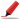Very frequently asked. Among first few questions in almost all interviews. Among Top 5 frequently asked questions. Frequently asked in Indian service companies (HCL,TCS,Infosys,Capgemini etc based on multiple feedback )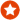Q1. Difference between == and .equals() ?Core Java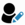Anonymous
Ans. "equals" is the method of object class which is supposed to be overridden to check object equality, whereas "==" operator evaluate to see if the object handlers on the left and right are pointing to the same object in memory.

x.equals(y) means the references x and y are holding objects that are equal. x==y means that the references x and y have same object.

Sample code:

String x = new String("str");
String y = new String("str");

System.out.println(x == y); // prints false
System.out.println(x.equals(y)); // prints true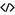Sample Code for equalsHelp us improve. Please let us know the company, where you were asked this question :LikeDiscussCorrect / Improvejava   string comparison   string   object class   ==    equals   object equality  operator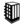Asked in 289 Companies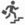basic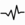frequent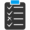Try 6 Question(s) TestRelated Questions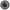What is the difference between = and == in Java ?How is == operator different for objects and primitive types ?What will be the output of following code

Integer x = 1;
Integer y = 2;
System.out.println(x == y);

What if you change 1 to "1" and Integer to String?Frequently asked in face to face interviews.Q2. Write a program to print fibonacci series.Core JavaAns. int count = 15;
int[] fibonacci = new int[count];
fibonacci = 0;
fibonacci = 1;
for(int x=2; x < count; x++){
fibonacci[x] = fibonacci[x-1] + fibonacci[x-2];
}

for(int x=0; x< count; x++){
System.out.print(fibonacci[x] + " ");
}Help us improve. Please let us know the company, where you were asked this question :LikeDiscussCorrect / Improveebay   fibonacci seriesAsked in 66 CompaniesbasicfrequentRelated QuestionsWhat are the steps to be performed while coding Junit with Mocking framework ?What is a Sequence File?What different level of logging you use while coding ?Have you ever encoded the response before sending it back from the service? If Yes , Which encoding was used ?What are the different type of encoding you have used ?How does encoding affect using Reader / writer classes or Stream classes in Java ?What is the difference between html encoding and url encoding ?Does spaces get's encoded in html encoding ?

Subscribe to Java News and Posts. Get latest updates and posts on Java from Buggybread.com